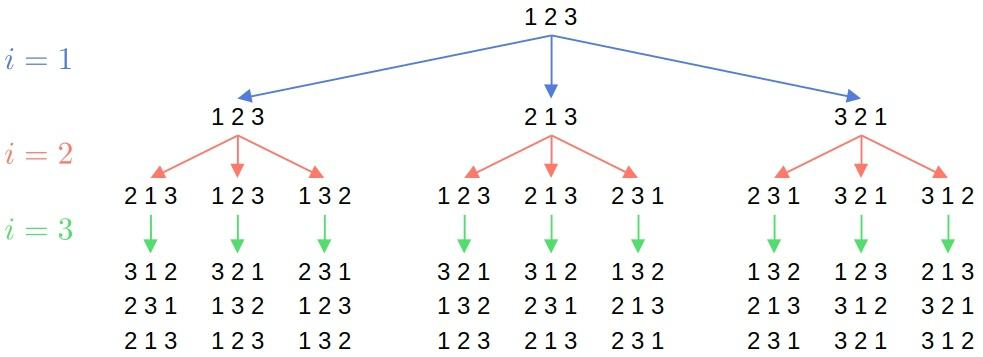## 1. Introduction

In this tutorial, we’ll learn how to shuffle an array.

## 2. Shuffling

In many applications, we have an arrayand need to shuffle it. For example, that’s the case when doing permutation tests to check feature importance in machine learning. Shuffling the arraymeans getting a random permutation of its elements.

We require all permutations to be equally likely.

## 3. Naïve Approach

We could first try something like this. We could iterate over, and for eachrandomly choose an indexand swapwith:This may come as an intuitive thing to do, but the issue is that not all permutations are equally likely.

In each of theiterations, we can choose any of theelements of. So, there areequally likely executions but onlydifferent permutations. Sinceisn’t divisible byfor any, we can’t spread outexecutions overpermutations evenly. So, some permutations are more likely than others.

### 3.1. Example

Here are all possible executions when the input is:As we see, permutationsoccur with the probabilityeach, whereas the rest with.

## 4. The Fisher-Yates Algorithm

Instead, we can use the Fisher-Yates algorithm. For each, it randomly selects  an indexfromto make a swap:Basically, whenever we chooseto swap with the current element, we discard it from participating in the swaps with. We achieve that by restricting the range for.

Therefore, the Fisher-Yates algorithm splits the array into two parts:and. We’ll call them the inactive and active parts. In theth iteration, the algorithm can choose only active elements for the exchange with. Afterward, the inactive part grows to include the element at theth position.

So, for each, there areactive elements to choose from. As a result, the total number of possible executions is:which matches the number of permutations. Since we can choose an element for a swap only once, there is only one way to get each permutation. Consequently, they’re all equally likely and occur with the probability of.

### 4.1. Example

Let’s say. Here’s a possible execution where | denotes the split:In the last step, there’s only one possibility for, so we stop and return.

### 4.2. Potential Issues

Random integer generation is at the core of the Fisher-Yates algorithm. If we can’t sample from a truly uniform distribution over, the algorithm will be biased toward some permutations. That can happen in two ways.

Let’s say we have a random number generatorthat returns integers fromtoinclusive (is usually the maximal integer). We can getlike this:For all the remainders to be equally likely,must be divisible by each possible, which we can’t assume to be the case. As some remainders are more likely than others, not alls we compute with the above formula are equally probable. However, the higher the, the less the discrepancy.

On the other hand, if we have a generator that returns random numbers from, we can getlike this:Since computers have finite precision,can’t return all real numbers from, so rounding won’t result in equally probable. Addingto the result of rounding does give us integers from the desired range, but not all are equally likely.